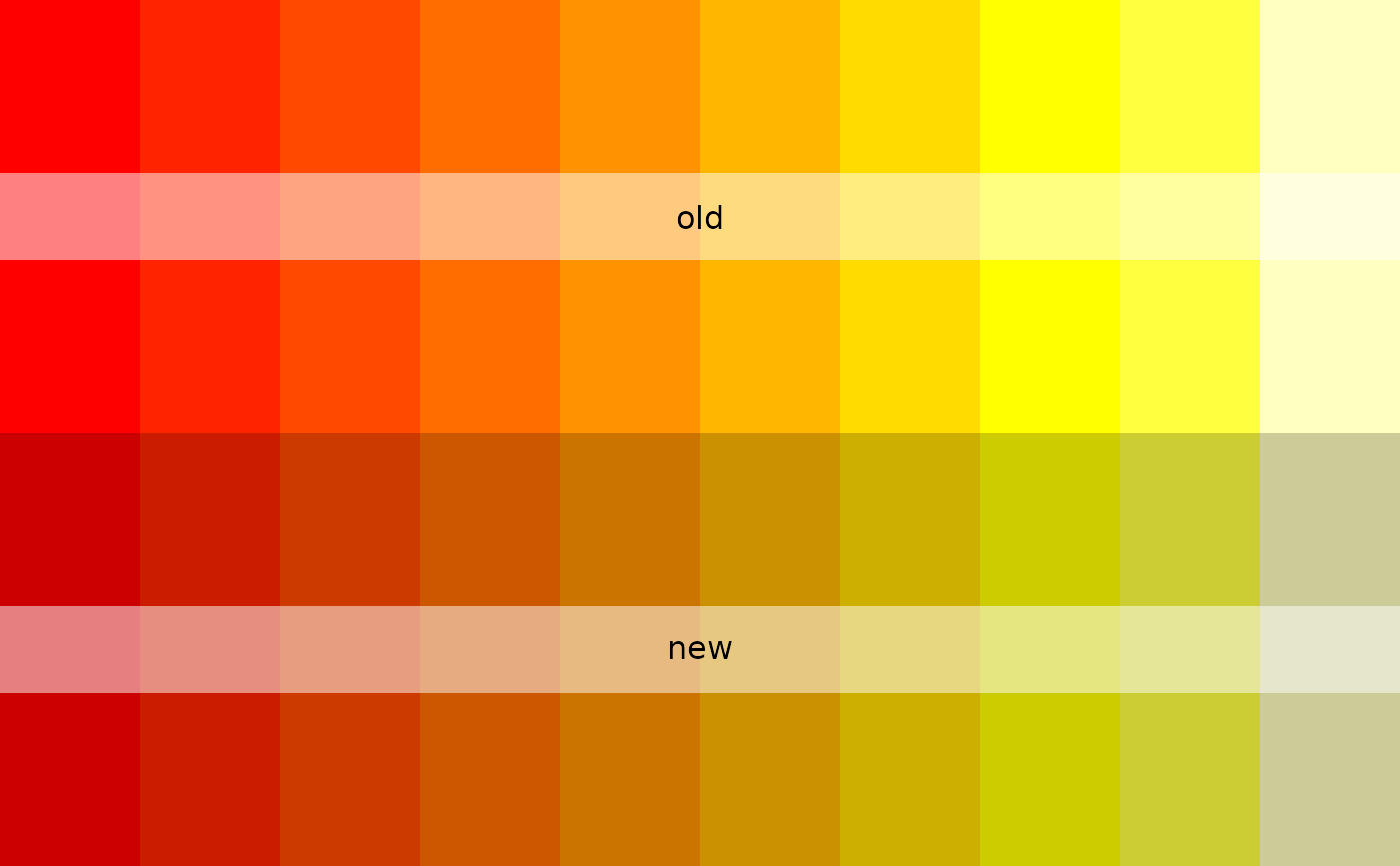Adjusts a given colour by lightening or darkening it by the specified amount (relative scale of -1 to 1). Adjustments are made in RGB space, for limitations of which see `?convertColor`

## Usage

``adjust_colours(cols, adj = 0, plot = FALSE)``

## Arguments

cols

A vector of `R` colours (for allowable formats of which, see `?col2rgb`).

A number between -1 and 1 determining how much to lighten (positive values) or darken (negative values) the colours.

plot

If `TRUE`, generates a plot to allow visual comparison of original and adjusted colours.

## Value

`osm_structures`, `?col2rgb`.

Other colours: `colour_mat()`

## Examples

``````cols <- adjust_colours (cols = heat.colors (10), adj = -0.2, plot = TRUE)# 'adjust_colours' also offers an easy way to adjust the default colour
# schemes provided by 'osm_structures'. The following lines darken the
# highway colour of the 'light' colour scheme by 20%
structures <- osm_structures (structures = c("building", "highway", "park"),
col_scheme = "light")
# Plot these structures:
bbox <- get_bbox (c (-0.13, 51.5, -0.11, 51.52))
if (FALSE) {
dat_B <- extract_osm_objects (key = "building", bbox = bbox)
dat_H <- extract_osm_objects (key = "highway", bbox = bbox)
dat_P <- extract_osm_objects (key = "park", bbox = bbox)
}
# These data are also included in the 'london' data of 'osmplotr'
osm_data <- list (dat_B = london\$dat_BNR,
dat_H = london\$dat_HP,
dat_P = london\$dat_P)
dat <- make_osm_map (structures = structures,
osm_data = osm_data,
bbox = bbox)
print_osm_map (dat\$map)
``````